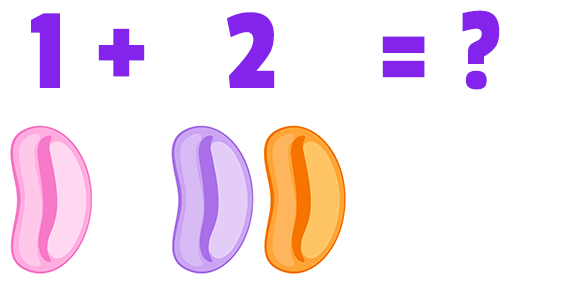# Add & Subtract Up to 5

Description: Fluently add and subtract numbers up to 5.

Question 1 (Short Answer, 10 points)Question 2 (Short Answer, 10 points)

3 + 2 = ?

Question 3 (Short Answer, 10 points)

4 - 1 = ?

Question 4 (Short Answer, 10 points)

1 + 3 = ?

Question 5 (Short Answer, 10 points)

1 - 1 = ?